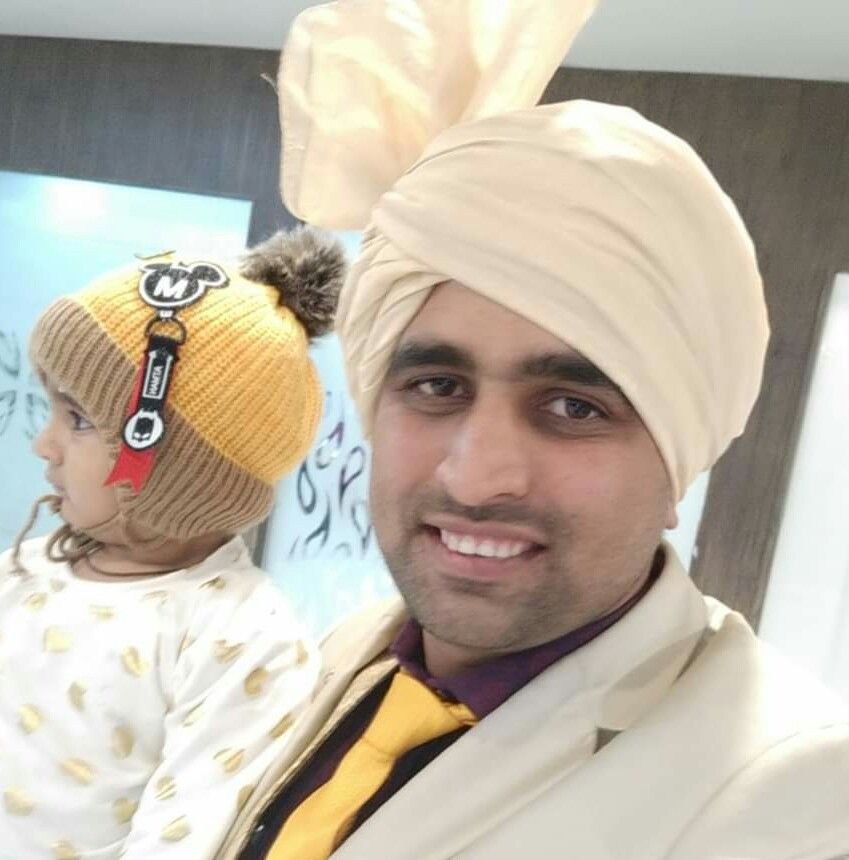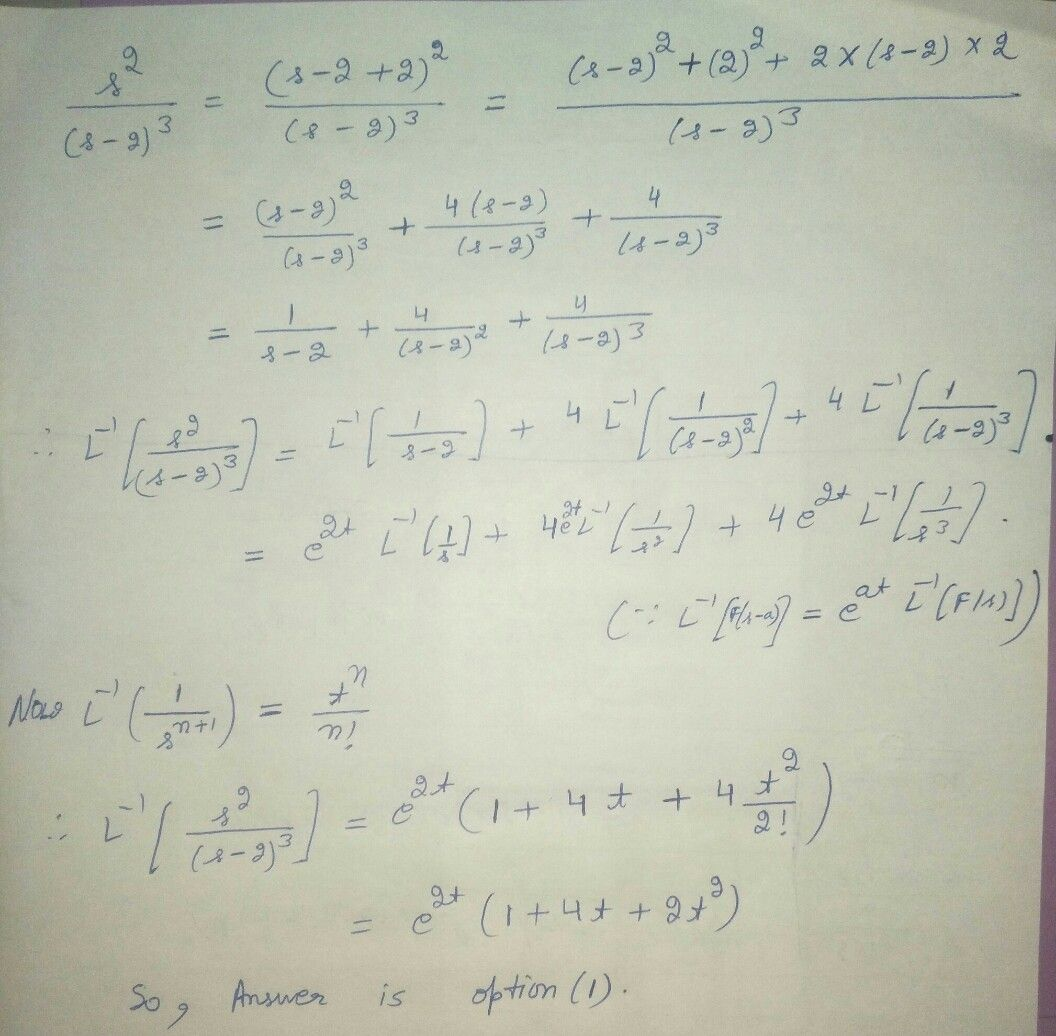Symbol
Problem$21$ $L^{-1}\left(\dfrac {s^{2}} {\left(s-2\right)^{3}}\right)=$ $1\right)$ $e^{2t}\left(1+4t+2t^{2}\right)$ $2\right)$ $e^{21}\left(1-4t+2t^{2}\right)$ $3\right)$ $e^{2t}\left(1-2t+4t^{2}\right)$ $4\right)$ $e^{-2t}\left(1-2t+4t^{2}\right)$
10th-13th grade
Other
Search count: 111
Question content
Laplace transforms chapter please solve
SolutionQanda teacher - AnoopDhill